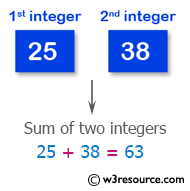﻿ Swift Basic Programming Exercise: Compute the sum of the two integers - w3resource# Swift Basic Programming Exercise: Compute the sum of the two integers

## Swift Basic Programming: Exercise-1 with Solution

Write a Swift program to compute the sum of the two integers. If the values are equal return the triple their sum.

Pictorial Presentation:Sample Solution:

Swift Code:

``````func triple_sum(a: Int, b: Int) -> Int {
if a == b
{
return (a + b) * 3
}
else
{
return a + b
}
}

print(triple_sum(a: 1, b: 2))
print(triple_sum(a: 3, b: 2))
print(triple_sum(a: 2, b: 2))
```
```

Sample Output:

```3
5
12
```

Swift Programming Code Editor:

Improve this sample solution and post your code through Disqus

What is the difficulty level of this exercise?

﻿